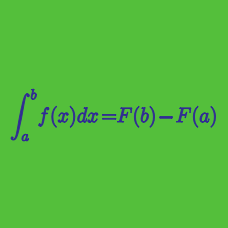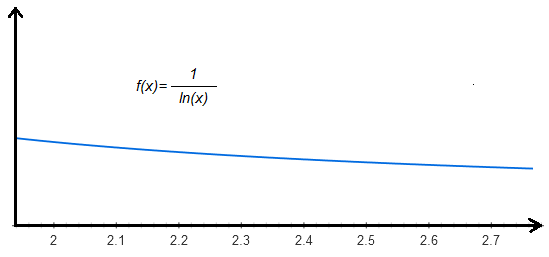Calculus

# Definite Integrals: Level 2 Challenges

Let $f(x)$ be a real-valued function continuous on $\left[0,2\right]$ such that $f(x)=f(2x)$ for all $x$. If

$\int_0^1 f(x) dx = 100,$

then what is the value of

$\int_0^2 f(x)dx ?$

Let $a,b,c$ be non-zero real constants such that $\displaystyle \int_{0}^3 (3ax^2+2bx+c) \, dx=\displaystyle \int_{1}^3 (3ax^2+2bx+c) \, dx.$ What is the value of $a+b+c$?

$\bigg \lfloor \;10 \times \displaystyle \int_2^e \! \frac {1}{\ln(x)} \, \mathrm{d}x\;\bigg \rfloor = \ ?$

Details and Assumptions:

• You may use the following approximations: $e \approx 2.718$, and $\ln(2) \approx 0.693$, and use the following graph of $f(x)= \frac {1}{\ln(x)}$.$\displaystyle \int_{0}^{12} \Bigl( \{x\}^2+\lfloor x \rfloor ^2\Bigr) \ dx = \ ?$

Details and assumptions:

• Every $x\in \mathbb{R}$ can be written as $x=\lfloor x \rfloor + \{x\}$.
• As usual, $\lfloor x \rfloor$ denotes greatest integer less than or equal to $x$.
• $\{x\}$ is the fractional part of $x$.

If $\int_0 ^ {3} f(x) \, dx = 2$, what is the value of

$\int_0^{3} \left( 3 - f(x) \right) \, dx ?$

×